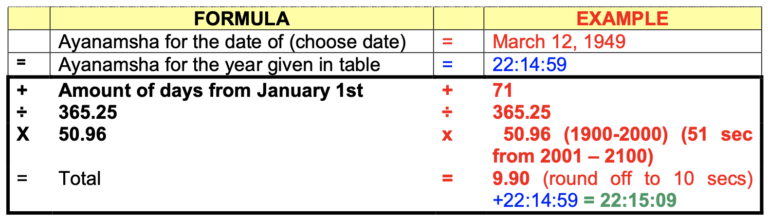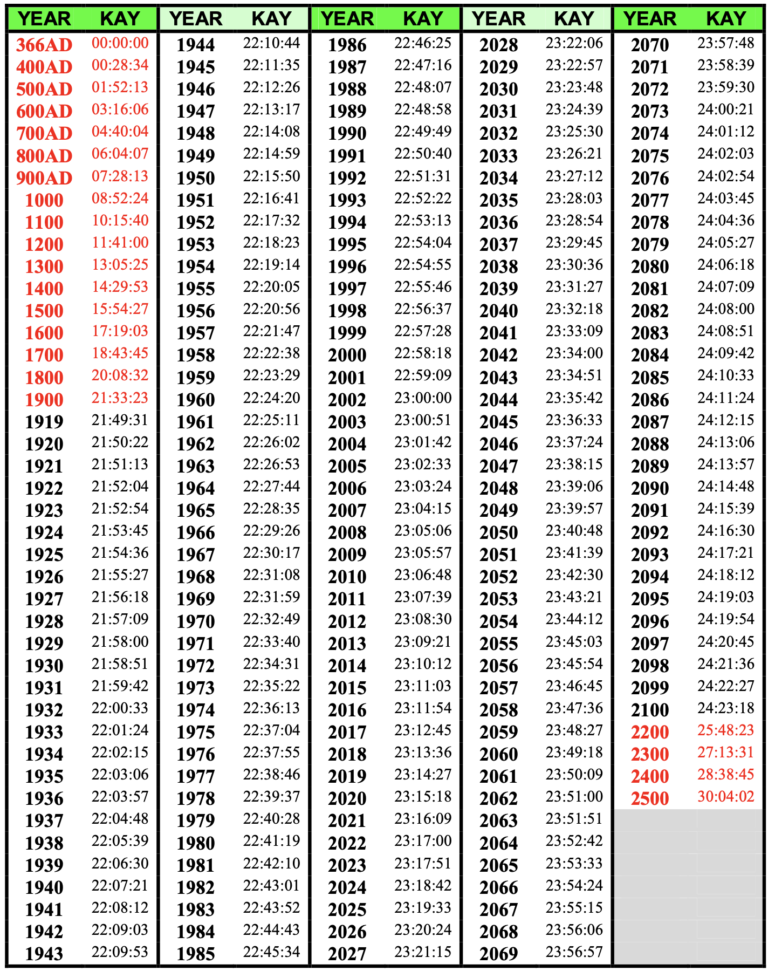12 Divisions

enquire@12divisions.com

Patterns of destiny & rhythms

# KAS Astrology Lessons

Krushnaji had written a series of lessons on KAS during 2000-2002 and he made them available to KAS Yahoo Group members. Mrs. Donna Quinn was kind enough to proof read the lessons and fix typos and grammar to make the lessons easily comprehensible. I am reproducing the KAS lessons here to support new KAS students. The only change I have made is to provide screen shots of charts from online KAS software which uses an improved KAS ayanamsa value than the one that students were using during 2000-2002.

Credits: All credits go to Krushnaji and Donnaji for providing this wealth of information.

## Lesson 6: Krushna's Ayanamsa Table

There are two systems of calculating the longitude of the planets, the Tropical Zodiac (Sayana) and the Sidereal Zodiac (Nirayana). The longitudinal differences between these two systems is known as the ayanamsa.

We use the Sidereal system, so the correct value on the ayanamsa is very important. The longitudes of the planets are dependent upon the ayanamsa as it will effect not only the longitude of the Ascendant, but the 16 divisional charts as well. The Vimshottari Dasha is calculated from the Moon’s longitudes and one second of difference may result in 1 to 3.6 hours difference in the dasha period, so a difference of one minute will be equal to 2.5 days to 9 days.

Different scientists and astrologers are of different opinions about this “overlapping” of both zodiacs. Both of them actually overlapped in 285AD, but since then, the accumulated ‘out of alignment’ difference between these two zodiacs has widened throughout the years to 23-24 degrees and is known as the “precession of the equinox”. Due to this precession, it moves at a rate of about one degree every 72 years. Thus, the starting point when the two zodiacs last coincided, has been given anywhere from 388 B.C to 498 A.D. The values can range anywhere from 46.3 seconds to 59.9 seconds per year.

I have chosen the starting date that both zodiacs coincided last, as 24th Feb 366 A.D.

For the year 1900, Krushna’s Ayanamsa is 21:33:23. The yearly speed of precession from 1900 to 2000 is 50.96. From the year 2001 to 2100, the yearly rate of precession is 51 seconds.

This will give the most satisfactory value of Krushna’s Ayanamsa.

### Using the formula chart:

In the following example on the next page, you will be able to calculate manually how to come to the proper ayanamsa value for whatever day you were born by following the template given in the formula chart, using March 12, 1949, below, as an example.

By checking the table “HOW TO FIND THE NUMBER DAY OF THE YEAR”, you can see that March 12th is day 71 from January 1st.

Over a period of one year or 365.25 days, the total precession is 00:00:51 or 51 seconds. Therefore 365.25 is the equivalent of 51 seconds and 71 is equivalent to how many seconds have gone by so far. The math now becomes: 71 / 365.25 * 51 seconds = 9.913758 seconds. Round this off to 10 seconds and add those 10 seconds to the ayanamsa value for the year 1949 that can be found in Krushna’s Ayanamsa Table on the next page. (see the Formula Chart below).

### Formula chart to use as a templateThe total value in BOLD lettering will be in seconds, so addition should also be in seconds. The final ayanamsha for the date of March 12, 1949 is 22:15:09

The precession for the year 1900 to 2000 may be taken as 50.96

For years 2000 to 2100, it is 51.00

For years 2100 to 2200, it is 51.05

For years 2200 to 2300, it is 51.08

For years 2300 to 2400, it is 51.14

Actually, the increase is approximately 0.044 seconds over a span of 100 years, so per year, it is increasing by a value of 0.00044 seconds per year.

In the table below, I will give my Ayanamsha Table. It starts off with the year 366AD (Feb 24th), then reverts to Jan 1st for all the other longitudes. Starting from the year 1919, it will give every consecutive year thereafter up until the year of 2100. From the years 2200-2500, it lists the ayanamsa in 100 year intervals. (marked in RED in table).

## Krushna's Ayanamsa Table